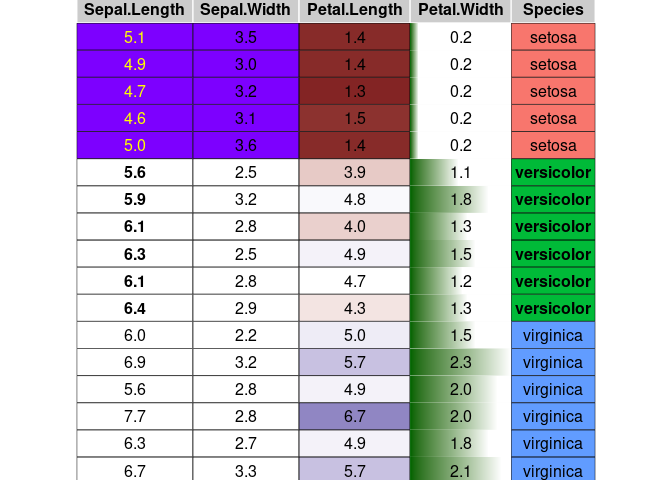# Introduction to condformat

Sergio Oller 2022-11-26

condformat renders a data frame in which cells in columns are formatted according to several rules or criteria.## Browse source code

Checkout the code and browse it at https://github.com/zeehio/condformat.

## How to install condformat:

### Dependencies

If you want to use the PDF output you will need the `xcolor` LaTeX package. Either use the full texlive distribution, or install `latex-xcolor` on Debian and derivatives.

### Package installation

• From CRAN:

``install.packages("condformat")``
• To install the latest development version:

``remotes::install_github("zeehio/condformat")``

## Example

``````data(iris)
library(condformat)
condformat(iris[c(1:5,70:75, 120:125),]) %>%
rule_fill_discrete(Species) %>%
rule_fill_discrete(c(Sepal.Width, Sepal.Length),
expression = Sepal.Width > Sepal.Length - 2.25,
colours = c("TRUE" = "#7D00FF")) %>%
rule_text_bold(c(Sepal.Length, Species), Species == "versicolor") %>%
rule_text_color(Sepal.Length,
expression = ifelse(Species == "setosa", "yellow", "")) %>%
rule_fill_bar(Petal.Width, limits = c(0, NA)) %>%
theme_grob(rows = NULL) %>%
condformat2grob()``````This example covers most of the condformat rules.

## Rules and output engine support

Rule HTML LaTeX gtable Excel
`rule_fill_discrete` X X X X
`rule_fill_gradient` X X X X
`rule_fill_gradient2` X X X X
`rule_text_color` X X X X
`rule_text_bold` X X X X
`rule_fill_bar` X X
`rule_css` X